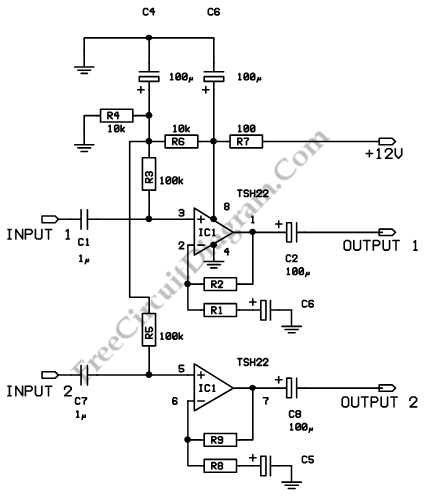# TSH22 Audio Line DriverThis is an audio line driver circuit using a TSH22. The advantages of this circuit are low distortion, exhibits a wide bandwidth and an output current sufficient to drive medium impedance loads at a high level modulation. Here is the circuit :This circuit uses two TSH22 that is used in non inverting mode. The gain of this cricuit can be set by resistors. This circuit only uses a single power supply with voltage between 8V and 30V. A phantom ground at Vcc/2 is made by two resistors (R4 and R6).

Following equations show the overall gain for frequency in the bandwidth :
Gain channel 1 = (R1 + R2)/R1
Gain channel 2 = (R8 + R9)/R8

[Source: STMicroelectronics Application Note]